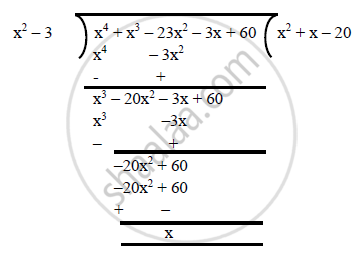# Find All the Zeroes of (X^4 + X^3 – 23x^2 – 3x + 60), If It is Given that Two of Its Zeroes Are Sqrt3 and –Sqrt3. - Mathematics

Find all the zeroes of (x^4 + x^3 – 23x^2 – 3x + 60), if it is given that two of its zeroes are sqrt3 and –sqrt3.

#### Solution

Let f(x) =x^4 + x^3 – 23x^2 – 3x + 60
Since sqrt3 and –sqrt3 are the zeroes of f(x), it follows that each one of (x – √3) and (x + √3) is a factor of f(x).
Consequently, (x – sqrt3) (x + sqrt3) = (x^2 – 3)is a factor of f(x).
On dividing f(x) by (x^2 – 3), we get:f(x) = 0
⇒ (x^2 + x – 20) (x^2 – 3) = 0
⇒ (x^2 + 5x – 4x – 20) (x^2 – 3)
⇒ [x(x + 5) – 4(x + 5)] (x^2 – 3)
⇒ (x – 4) (x + 5) (x – sqrt3) (x + sqrt3) = 0
⇒ x = 4 or x = -5 or x = sqrt3 or x = -sqrt3
Hence, all the zeroes are √3, -√3, 4 and -5.

Concept: Relationship Between Zeroes and Coefficients of a Polynomial
Is there an error in this question or solution?